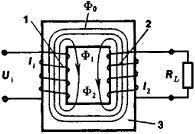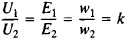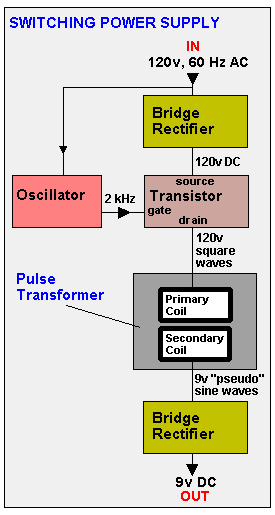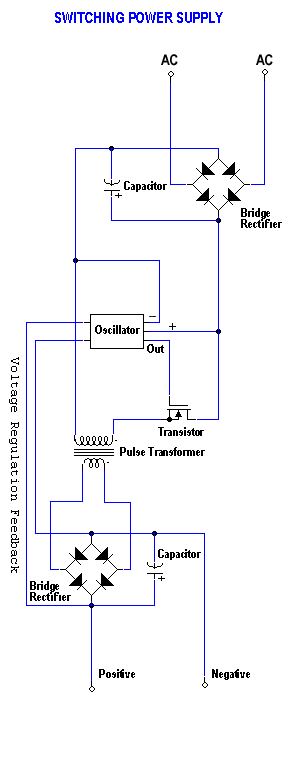# transformer

(redirected from AC transformer)
Also found in: Dictionary, Thesaurus, Medical.

## transformer,

electrical device used to transfer an alternating current or voltage from one electric circuit to another by means of electromagnetic inductioninduction,
in electricity and magnetism, common name for three distinct phenomena. Electromagnetic induction is the production of an electromotive force (emf) in a conductor as a result of a changing magnetic field about the conductor and is the most important of the
. The simplest type of transformer consists of two coils of wire, electrically insulated from one another and arranged so that a change in the current in one coil (the primary) will produce a change in voltage in the other (the secondary). In many transformers the coils are wound on a core made of a material with high magnetic permeability; this intensifies the magnetic field induced by the current in the primary, increasing the transformer's efficiency. Neglecting power losses (which are made small by careful design), the ratio of primary voltage to secondary voltage is the same as the ratio of the number of turns in the primary coil to the number of turns in the secondary coil. The primary and secondary currents are in inverse proportion to the number of turns in the coils. The primary and secondary impedancesimpedance,
in electricity, measure in ohms of the degree to which an electric circuit resists the flow of electric current when a voltage is impressed across its terminals.
are in the same ratio as the squares of the numbers of turns in the primary and secondary coils. For example, if a 10-volt, 2-ampere alternating current were to flow through a 10-turn primary of a transformer, theoretically a 20-turn secondary would exhibit a 20-volt, 1-ampere alternating current, with the output impedance four times as great as the input impedance. Transformers are frequently classified according to their uses; the details of construction depend on the intended application. Power transformers are generally used to transmit power at a constant frequency. Audio transformers are designed to operate over a wide range of frequencies with a nearly flat response, i.e., a nearly constant ratio of input to output voltage. Radio frequency (RF) transformers are designed to operate efficiently within a narrow range of high frequencies.

## Transformer

A device that carries electrical current from one source to another, usually with an increase or decrease in voltage.

## Transformer

a static device (with no moving parts) for transforming the magnitude of an AC voltage. The operation of a transformer is based on the phenomenon of electromagnetic induction.

A transformer consists of a primary winding, one or more secondary windings, and a ferromagnetic core, or magnetic circuit, which is usually closed (see Figure 1). All the windings are located on the magnetic circuit and are inductively linked (seeMUTUAL INDUCTANCE). Sometimes part of the primary winding serves as the secondary, or vice versa; such transformers are called autotransformers. The terminals of the primary (the transformer input) are connected to a source of AC voltage, and those of the secondary (the output) are connected to the consumers. The alternating current in the primary produces an alternating magnetic flux in the magnetic circuit. In real transformers, part of the magnetic flux is closed outside the magnetic circuit, thereby forming a leakage flux; in high-quality transformers, however, the leakage fluxes are small compared to the mutual flux (the flux in the magnetic circuit).Figure 1. Simple electrical transformer: (1) and (2) primary and secondary windings, respectively, with w1 and w2 turns; (3) core; (Φ0) mutual flux; (Φ1) and (Φ2) leakage fluxes; (l1) and (l2) currents in the primary and secondary windings; (U1) voltage on the primary winding; (RL) load resistance

The mutual flux Φ0 produces the electromotive forces (emf’s) e1 and e2 in the primary and secondary windings, respectively: e1 = – w1dΦ0/dt, and e2 = – w2dΦ0/dt, where w1 and w2 are the number of turns on the corresponding windings. The ratio e1/e2 = w1/w2 = k is called the turn ratio. The voltages, currents, and emf’s (disregarding the emf’s induced by the leakage fluxes) are associated by the relationships

u1 + e1 = i1r1

and

u2 + i2r2 = e2

where r1 and r2, u1 and u2, i1 and i2 are the resistances, voltages, and currents of the windings. If the voltage u1 applied to the primary is sinusoidal, then the magnetic flux Φ0 and the emf’s e1 and e2 will also be sinusoidal. Therefore, in the analysis of the operation of transformers it is convenient to consider the effective values of the emf’s E1 and E2, of the voltages U1 and U2, and of the currents I1 and I2. For the no-load condition (secondary open), disregarding the resistance of the primary and assuming that I2 = 0, we will have U1 + E1 = 0 and U2 = E2, that is (without regard to sign),The mutual magnetic flux under no-load conditions is produced by the relatively small magnetizing current (the no-load current I0) in the primary. If the transformer is loaded (the secondary is connected to a load, and current is flowing through it), the magnetomotive force of the secondary (the product I2w2) is compensated by a corresponding increase in the magnetomotive force of the primary (I1w1I0w1), and the value of the mutual magnetic flux remains virtually the same as for the no-load condition (that is, the condition U1 + E1 = 0 is preserved). Thus, by neglecting the no-load current we obtain I1w1I2w2.

Transformers were first used in 1876 by P. N. lablochkov in electric lighting circuits. In 1890, M. O. Dolivo-Dobrovol’skii developed a three-phase transformer. The subsequent development of transformers produced improvements in design, increases in power and efficiency, and improvement of winding insulation. As of the mid-1970’s, many types of transformers had become commonplace in various fields of technology.

Power transformers are the main type of transformer, and among these the most representative group is the double-wound power transformers, which are used on power lines: Power transformers raise the voltage of the current generated at electric power plants from 10–15 kilovolts (kV) up to 220–750 kV, thus making possible the transmission of electric power over aerial transmission lines for several thousand kilometers. At locations where electric power is consumed, the high voltage is stepped down to low voltages (220 V, 380 V, and so on) by other power transformers. The repeated transformation of the electric power requires a large number of power transformers, and therefore the total power of the transformers in the system is several times that of the sources and power users. High-capacity power transformers have an efficiency of 98–99 percent. Their windings are generally made of copper, and their magnetic circuits of cold-rolled sheets of electrical steel 0.5–0.35 mm thick that have high magnetic permeability and low hysteresis and eddy-current losses. The magnetic circuit and windings of power transformers are usually mounted in a tank filled with mineral oil, which is used to insulate and cool the windings. Such transformers—called oil-filled transformers—are generally located in the open, which requires improved insulation of the leads and hermetic sealing of the tank. Transformers without oil cooling are called dry transformers. For better heat dissipation, power transformers are equipped with a tubular radiator around which the air or, in some cases, water circulates. In lightning-proof transformers the windings are designed so as to avoid the appearance of dangerous voltages across the insulation. Sometimes two or three transformers are connected in series. In many cases transformers with load tap changing are used. Among the dry transformers, low-power transformers with a large number of secondary windings (multiwinding transformers) make up a broad class. They are frequently used in radio engineering apparatus and automatic systems.

In addition to power transformers, there are many types designed to measure high voltages and currents (for example, instrument transformers, voltage transformers, and current transformers), to reduce the level of interference on wire communication lines (negative booster transformers), to convert a sine voltage to a pulse voltage (peaking transformers), to convert current and voltage pulses (peak transformers), to isolate an AC current component, and to decouple and match parts of an electric circuit.

Radio-frequency transformers are used to transform high-frequency voltages; they are made with magnetodielectric magnetic circuits or without a magnetic circuit. In radio transmitters the power of such transformers reaches several hundred kilowatts.

### REFERENCES

Petrov, G. N. Elektricheskie mashiny, 3rd ed., part 1. Moscow, 1974.
Vol’dek, A. I. Elektricheskie mashiny. Leningrad, 1974.

V. S. KHVOSTOV

## transformer

[tranz′fȯr·mər]
(electromagnetism)
An electrical component consisting of two or more multiturn coils of wire placed in close proximity to cause the magnetic field of one to link the other; used to transfer electric energy from one or more alternating-current circuits to one or more other circuits by magnetic induction.

## transformer

A device with two or more coupled windings, used to convert a supply of electric power at one voltage to another voltage.

## transformer

a device that transfers an alternating current from one circuit to one or more other circuits, usually with an increase (step-up transformer) or decrease (step-down transformer) of voltage. The input current is fed to a primary winding, the output being taken from a secondary winding or windings inductively linked to the primary

## transformer

A device that is mostly used to change the voltage in an alternating current (AC); however, it can also be used to maintain the same voltage but act as an electrical isolator. The most common type is the laminated core transformer found in power supplies. Made of steel laminations wrapped with two coils of wire, the ratio of windings between the "primary" input coil and the "secondary" output coil determines the voltage change. For example, if the primary has 1,000 windings and the secondary 100, an input of 120 volts is changed to 12v.

Via ElectroMagnetic Induction
There are numerous transformer architectures, and they span the size gamut. Small ones are used in the myriad black boxes that plug into the wall and create low DC voltage for every electronic gadget, while transformers weighing tons are used to transmit 50,000 volts of AC over the nation's power grid. However, they all work via electromagnetic induction. The changing current in the primary coil induces a voltage across the secondary coil.

Switching Power Supplies
The greater the current needed to power the device, the thicker the wire in the coils and the larger the transformer. However, if a high frequency is used, the number of windings can be reduced to make the transformer small. To accomplish this, the incoming voltage is converted to DC (rectified), and a high-frequency oscillator pulses a transistor that passes the rectified voltage as square waves into a "pulse transformer." The on/off DC pulses cause the changing current in the primary coil just the same as AC does. This square wave generation turns a power supply into a "switching power supply." See power adapter, power supply and wall wart.

The Switching Power SupplyIn order to reduce the number of windings in transformer coils, a high frequency pulse transformer is used. This is a hypothetical example; voltages and frequencies vary. For example, the oscillator can generate frequencies from 1 kHz to 200 kHz. Following is a simplified circuit diagram of this power supply.Site: Follow: Share:
Open / Close